# Collection of FRACTAL ART Photos, Illustrations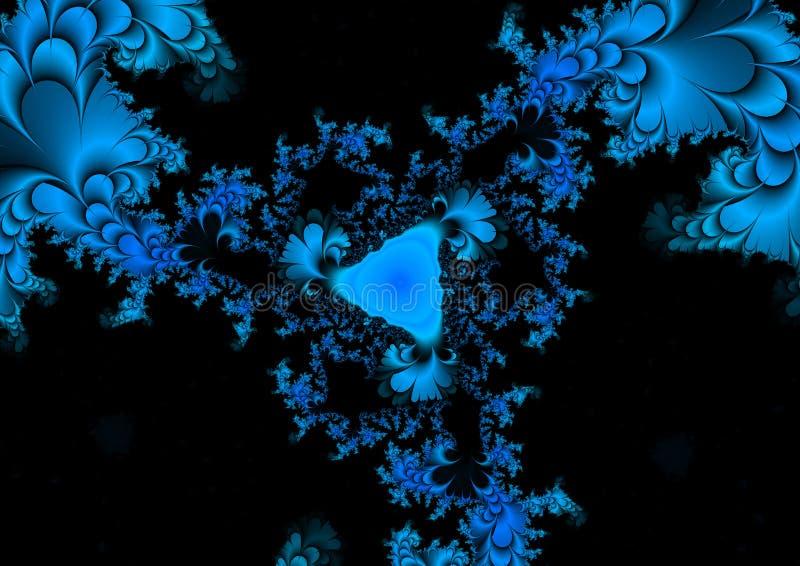Fractal background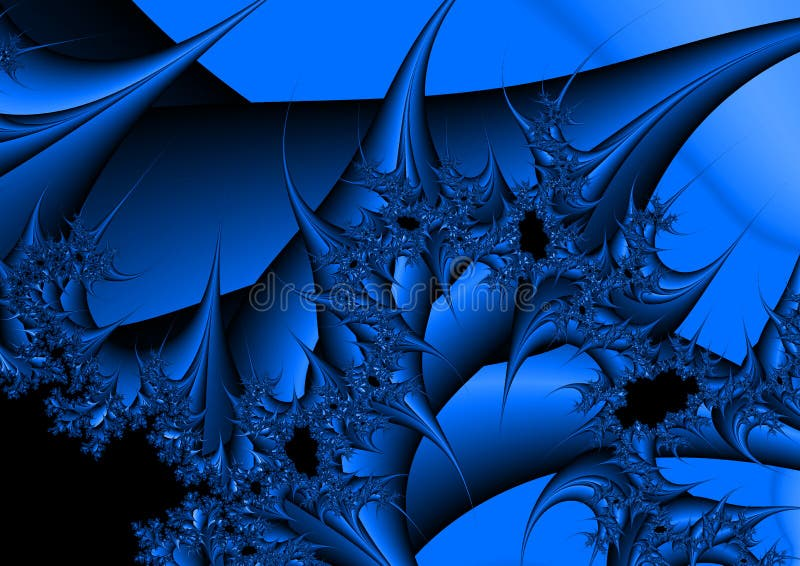Fractal background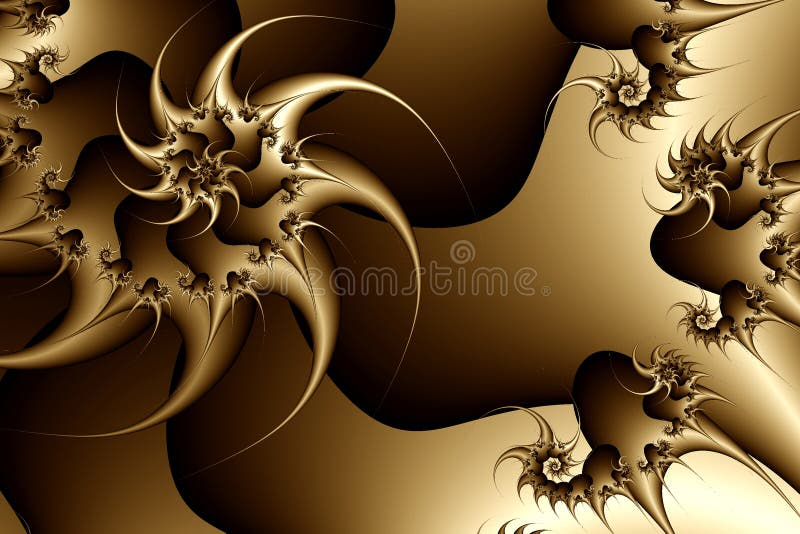Fractal texture, sepia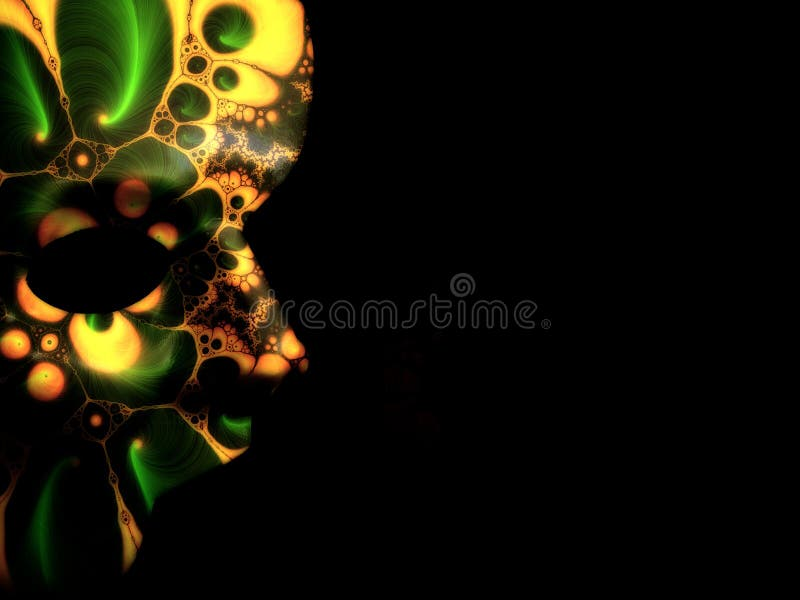Fractal Mask 2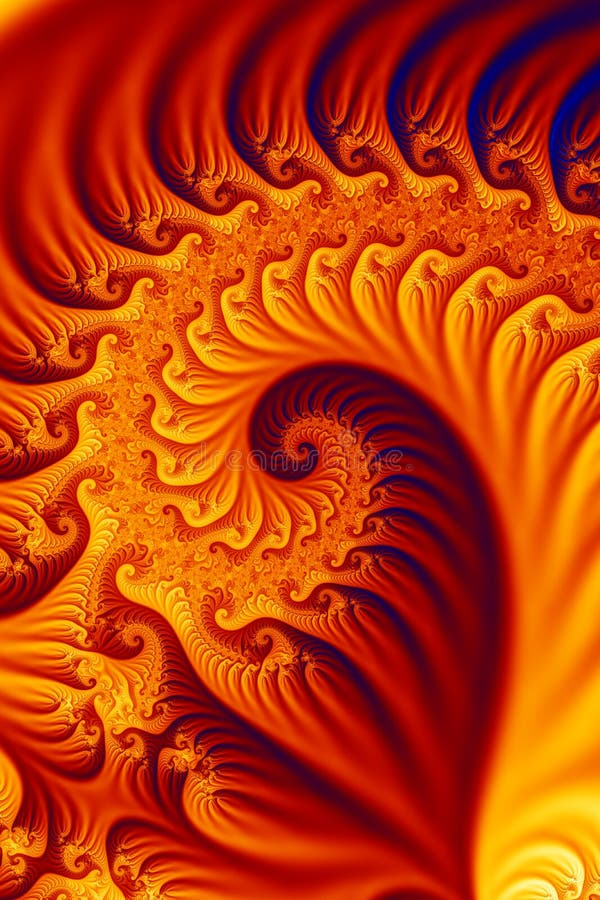Fiery fractal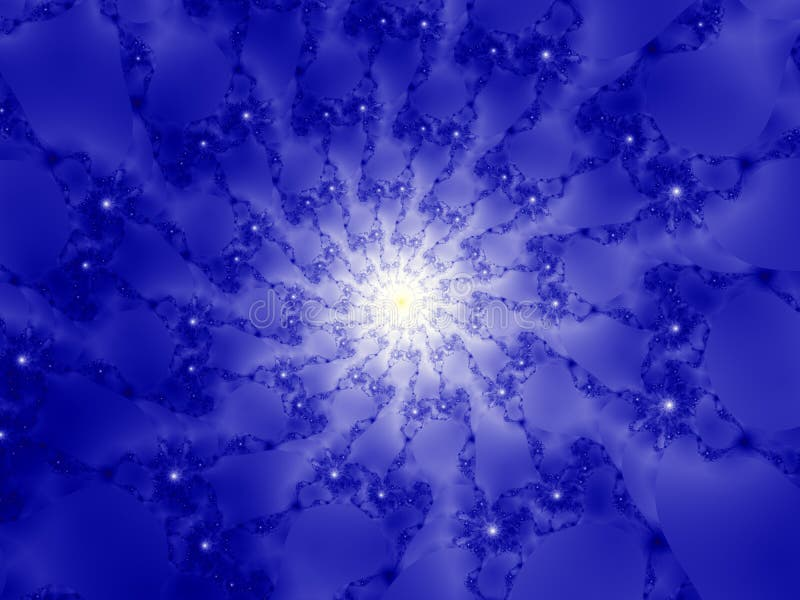Fractal birth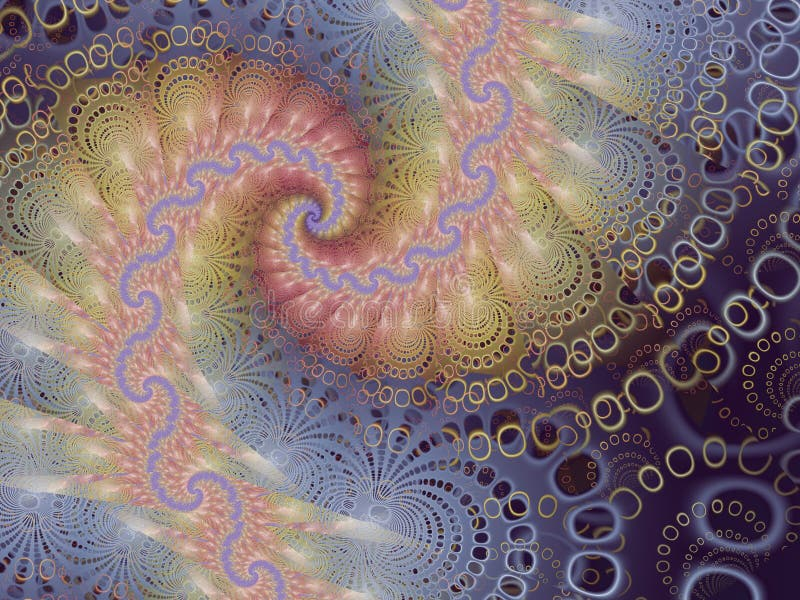Bubbly Fractal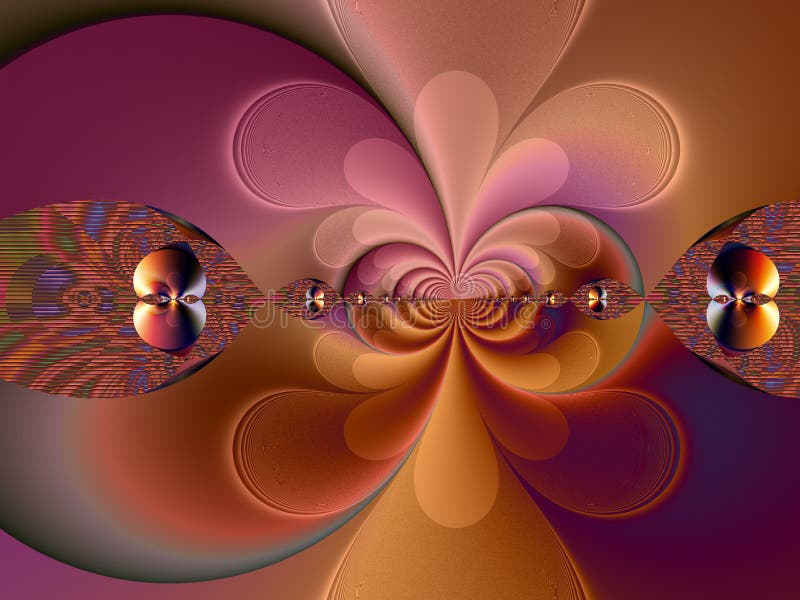60's Style Fractal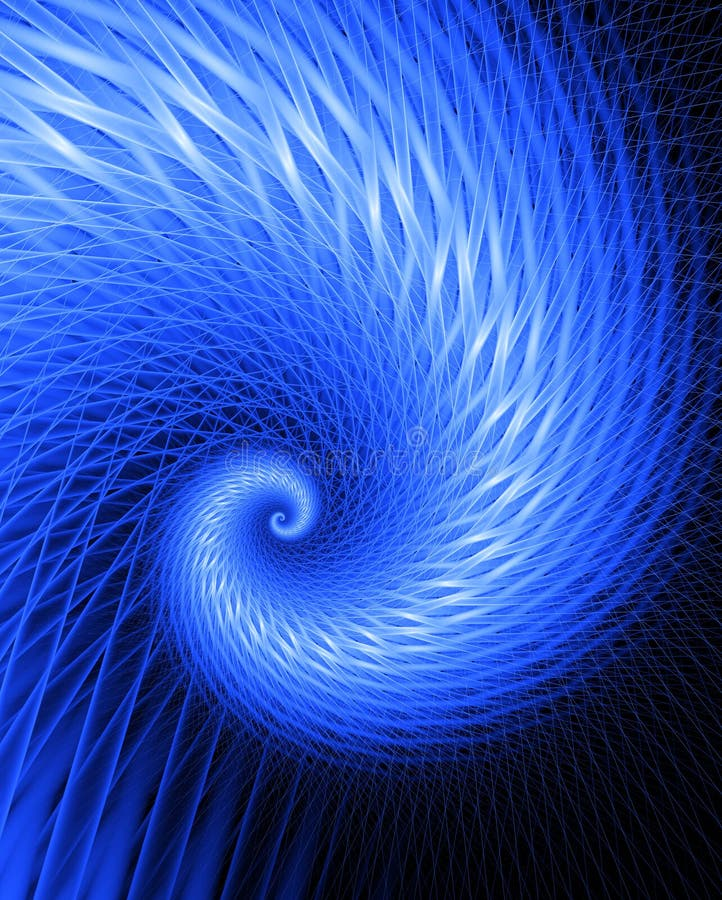Fractal Frenzy - Cool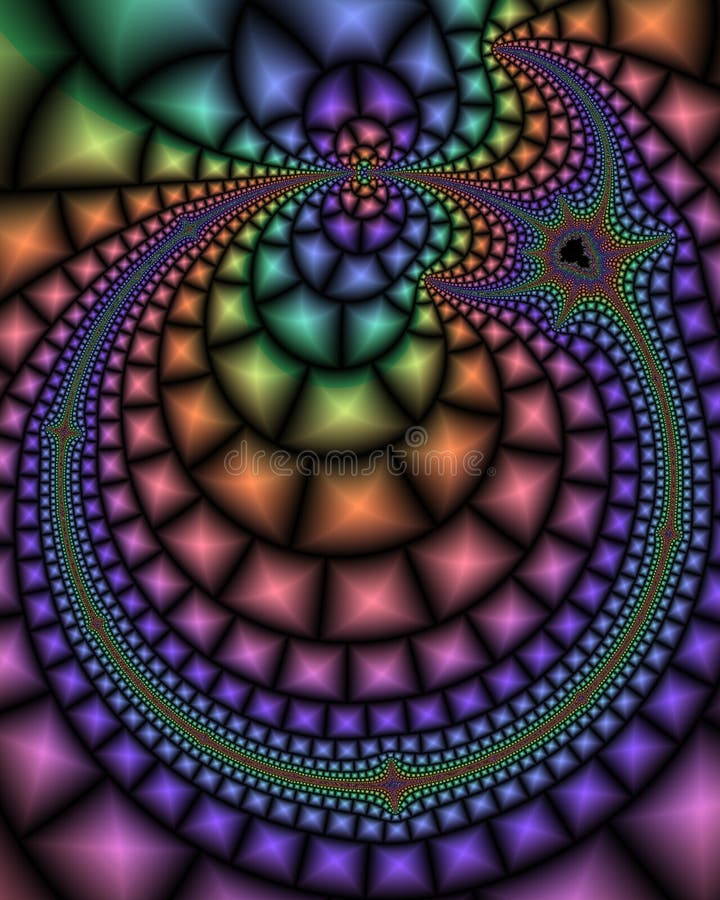Paisley FractalFushia Satin FractalGolden silk fractalPurple Ambrosia FractalColorful Teardrop Spiral - Fractal ImageFractal - Radiation SpiralRepeating Hearts - Fractal ImageBlack and Gold FractalFlame Fractal TurbineFractal abstract designFractalFractal HaloFractal BorderFire Fractal DesignAbstract FractalFractal SkullFractal yin yangFractal treeFractal Flower FusionBlue grid fractalPsychedelic fractalGalaxy FractalSolid FractalChristmas Lights - fractal imageFractal backgroundGolden FractalVibrant FractalHot lava fractalFractal SwirlFractal Ice CrystalFractal X-RayFire FractalInfinite Christmas Balls - Fractal ImageAlien julia fractal flameBubbly fractalColorfull spiral fractalFractal WaveFractal spiralMatter Splash FractalSpiky fractalShell fractalFractal HurricaneFractal SnowFractal_09aFractal Background (09d)Fractal_12L1Fractal light show frameFractal_09MBlue Spiral Wave Fractal SwirlFractal squares in spiralDance of Lights (fractal_02f)Fractal Brain (29a)Fractal galaxies (28a)Purple Flower Blossoms FractalSymmetrical Gold Fractal CrownFractal Flower Garden PetalsFractal LinesFractal flowerCosmic Fractal, (12s)Dark FractalBlue glowing fractalFractal cityAbstract fractal flame ballFire Ball Fractal Spiral FlameAlien Swirls Fractal TextureAlien Swirls Fractal Texture 2Pearls Lace Fractal Jewelry 2Pearls Lace Fractal NecklaceAlien Throne Bizarre FractalBizarre White Fractal NecklacePearls Lace Fractal Jewelry